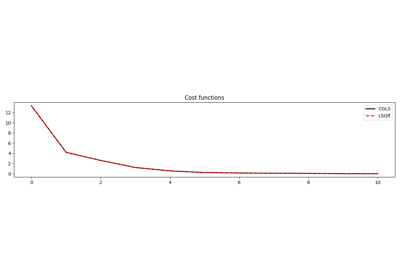# pylops.optimization.solver.lsqr¶

pylops.optimization.solver.lsqr(Op, y, x0, damp=0.0, atol=1e-08, btol=1e-08, conlim=100000000.0, niter=10, calc_var=True, show=False, callback=None)[source]

LSQR

Solve an overdetermined system of equations given an operator Op and data y using LSQR iterations.

Parameters: Op : pylops.LinearOperator Operator to invert of size $$[N \times M]$$ y : np.ndarray Data of size $$[N \times 1]$$ x0 : np.ndarray, optional Initial guess damp : float, optional Damping coefficient atol, btol : float, optional Stopping tolerances. If both are 1.0e-9, the final residual norm should be accurate to about 9 digits. (The final x will usually have fewer correct digits, depending on cond(A) and the size of damp.) conlim : float, optional Stopping tolerance on $$cond(\mathbf{Op})$$ exceeds conlim. For square, conlim could be as large as 1.0e+12. For least-squares problems, conlim should be less than 1.0e+8. Maximum precision can be obtained by setting atol = btol = conlim = 0, but the number of iterations may then be excessive. niter : int, optional Number of iterations calc_var : bool, optional Estimate diagonals of $$(\mathbf{Op}^H\mathbf{Op} + \epsilon^2\mathbf{I})^{-1}$$. show : bool, optional Display iterations log callback : callable, optional Function with signature (callback(x)) to call after each iteration where x is the current model vector x : np.ndarray Estimated model of size $$[M \times 1]$$ istop : int Gives the reason for termination 0 means the exact solution is $$\mathbf{x}=0$$ 1 means $$\mathbf{x}$$ is an approximate solution to $$\mathbf{y} = \mathbf{Op}\mathbf{x}$$ 2 means $$\mathbf{x}$$ approximately solves the least-squares problem 3 means the estimate of $$cond(\bar{\mathbf{Op}})$$ has exceeded conlim 4 means $$\mathbf{y} - \mathbf{Op}\mathbf{x}$$ is small enough for this machine 5 means the least-squares solution is good enough for this machine 6 means $$cond(\bar{\mathbf{Op}})$$ seems to be too large for this machine 7 means the iteration limit has been reached r1norm : float $$||\mathbf{r}||_2^2$$, where $$\mathbf{r} = \mathbf{y} - \mathbf{Op}\mathbf{x}$$ r2norm : float $$\sqrt{\mathbf{r}^T\mathbf{r} + \epsilon^2 \mathbf{x}^T\mathbf{x}}$$. Equal to r1norm if $$\epsilon=0$$ anorm : float Estimate of Frobenius norm of $$\bar{\mathbf{Op}} = [\mathbf{Op} \; \epsilon \mathbf{I}]$$ acond : float Estimate of $$cond(\bar{\mathbf{Op}})$$ arnorm : float Estimate of norm of $$cond(\mathbf{Op}^H\mathbf{r}- \epsilon^2\mathbf{x})$$ var : float Diagonals of $$(\mathbf{Op}^H\mathbf{Op})^{-1}$$ (if damp=0) or more generally $$(\mathbf{Op}^H\mathbf{Op} + \epsilon^2\mathbf{I})^{-1}$$. cost : numpy.ndarray, optional History of r1norm through iterations

Notes

Minimize the following functional using LSQR iterations :

$J = || \mathbf{y} - \mathbf{Opx} ||_2^2 + \epsilon^2 || \mathbf{x} ||_2^2$

where $$\epsilon$$ is the damping coefficient.

  Paige, C. C., and Saunders, M. A. “LSQR: An algorithm for sparse linear equations and sparse least squares”, ACM TOMS, vol. 8, pp. 43-71, 1982.

## Examples using pylops.optimization.solver.lsqr¶CGLS and LSQR Solvers